##### Actions

(diff) ← Older revision | Latest revision (diff) | Newer revision → (diff)

on a Riemann surfaceA rule which associates to each local parameter(cf. Local uniformizing parameter) mapping a parametric neighbourhood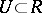into the extended complex plane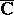(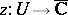), a function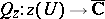such that for any local parametersand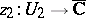withnon-empty, the following holds in this intersection:(1)

here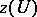is the image ofinunder. A quadratic differential is often denoted by the symbol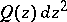, to which is attributed the invariance with respect to the choice of the local parameter, as indicated by (1). In other words, a quadratic differential is a non-linear differential of type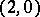on a Riemann surface.

The functionsentering into the definition of a quadratic differential are ordinarily assumed to be measurable or even analytic. In the latter case the quadratic differential is called analytic. A point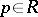is called a zero (or pole) ofof orderif for each local parameterthe functionhas a zero (or pole) of orderat. The zeros and poles of a quadratic differential are called critical points of it. The zeros and simple poles are called finite critical points and their totality is denoted by. The set of all poles of order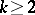is denoted by. If a curve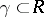has a tangent at each of its pointswith respect to the parameter, with tangent vector, and(2)

thenis said to be positive, and one writes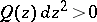, on the curve. If (2) holds with thesign replaced by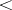, thenis called negative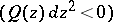on. Each maximal regular curve onfor which(or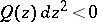) is called a trajectory (or orthogonal trajectory) of the quadratic differential.

A quadratic differentialdefined on a finite Riemann surfacebelongs toif the boundary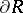ofis either empty or consists of a finite number of points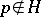and arcson each of whichis regular and positive or negative. If, furthermore,is empty or ifis regular and positive on, thenis called a positive quadratic differential on the Riemann surface. The metric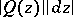, called a-metric, is single-valued onand invariant with respect to the choice of the local parameter.

In some neighbourhoodof any point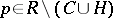, the function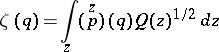is regular, single-valued and univalent for each choice of the sign of the integrand; furthermore, each maximal arc of a trajectory (or orthogonal trajectory) ofis converted underinto a horizontal (or vertical) line interval. Therefore, through each pointpasses a trajectory which is either an open arc or a Jordan curve on. The topological and conformal structures of the family of trajectories in a small neighbourhood of each critical pointare completely classified in their dependence on the order of the critical pointand (ifis a pole of the second order and) on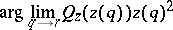(see Local structure of trajectories). A description of the global structure of trajectories is known for finite Riemann surfaces and has many important applications (see also ).

O. Teichmüller has investigated the role of quadratic differentials in the theory of extremal conformal and quasi-conformal mapping and in the solution of moduli problems of Riemann surfaces (see ). He formulated a principle according to which certain quadratic differentials can be associated with extremal problems in the geometric theory of functions, where to each type of extremal problem correspond specific singularities of the quadratic differential (poles), and the geometric properties of the solution are related in a suitable fashion to the structure of the trajectories of the quadratic differential. Inequalities for the coefficients of univalent functions (cf. Univalent function) have been proved in terms of quadratic differentials. A more general inequality for the coefficients of univalent functions in families of domains distributed on a finite Riemann surface is called the general coefficient theorem and is a concrete realization of the Teichmüller principle for a wide class of problems (see , ). The Teichmüller principle also enables one to establish a special coefficient theorem and to solve a large number of concrete extremal problems (see , ).

How to Cite This Entry: